# Intermediate Maths solutions for Matrices Exercise 3(h)

Inter Maths 1A text book Exercise 3(h) Matrices solutions are given.

These solutions are very easy to understand.

Please study the text book lesson Matrices very well.

Observe the example problems and solutions given in the text book.

Observe the given solutions and try them in your own method.

You can see the solutions for text book Maths 1A

Functions

Exercise 1(a)

Exercise 1(b)

Exercise 1(c)

Mathematical Induction

Exercise 2(a)

Matrices

Exercise 3(a)

Exercise 3(b)

Exercise 3(c)

Exercise 3(d)

Exercise 3(e)

Exercise 3(f)

Exercise 3(g)

Exercise 3(h)

Exercise 3(i)

Exercise 4(a)

Exercise 4(b)

Product of Vectors

Exercise 5(a)

Exercise 5(b)

Exercise 5(c)

Trigonometric Ratios up to Transformations

Exercise 6(a)

Exercise 6(b)

Exercise 6(c)

Exercise 6(d)

Exercise 6(e)

Exercise 6(f)

Trigonometric Equations

Exercise 7(a)

Inverse Trigonometric Equations

Exercise 8(a)

Hyperbolic Functions

Exercise 9(a)

Properties of Triangles

Exercise 10(a)

Exercise 10(b)

1B Maths solutions

Locus

Exercise 1(a)
Transformation of axes

Exercise 2(a)
Threedimensional Coordinates

Exercise 5(a)
Exercise 5(b)
Direction Cosines abd Direction Ratios

Exercise 6(a)
The Plane

Exercise 7(a)
Limits and Continuity

M

# Inter Maths Solutions for Matrices Exercise 3(h)

Matrices – Exercise 3(h)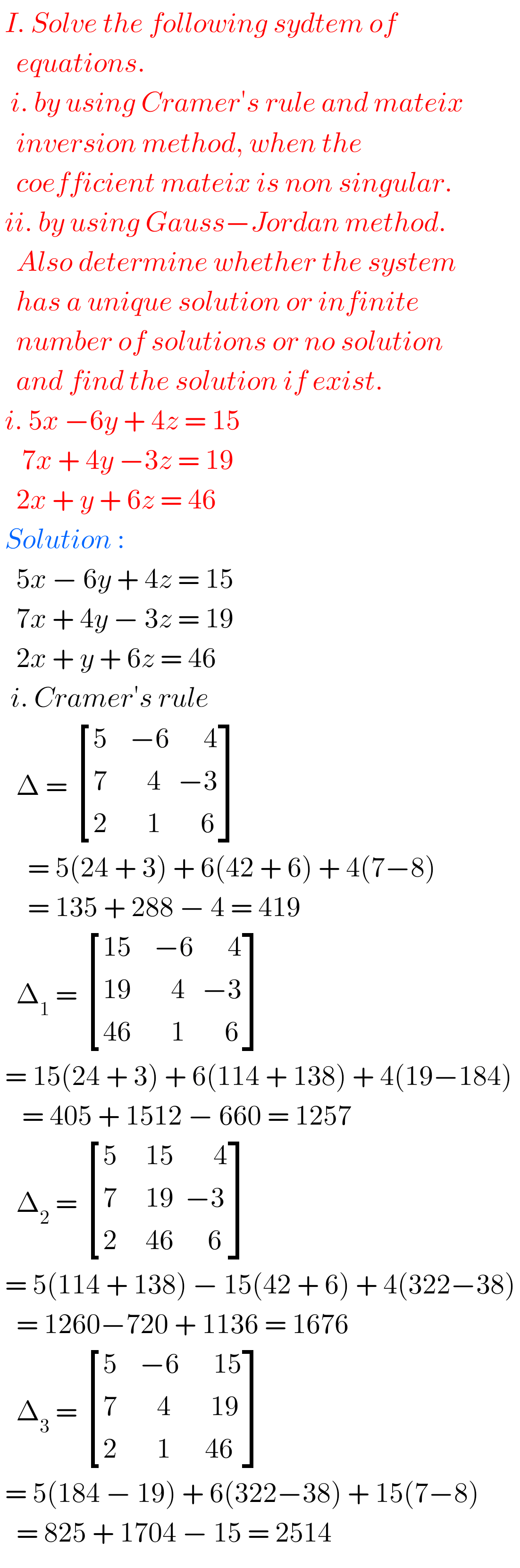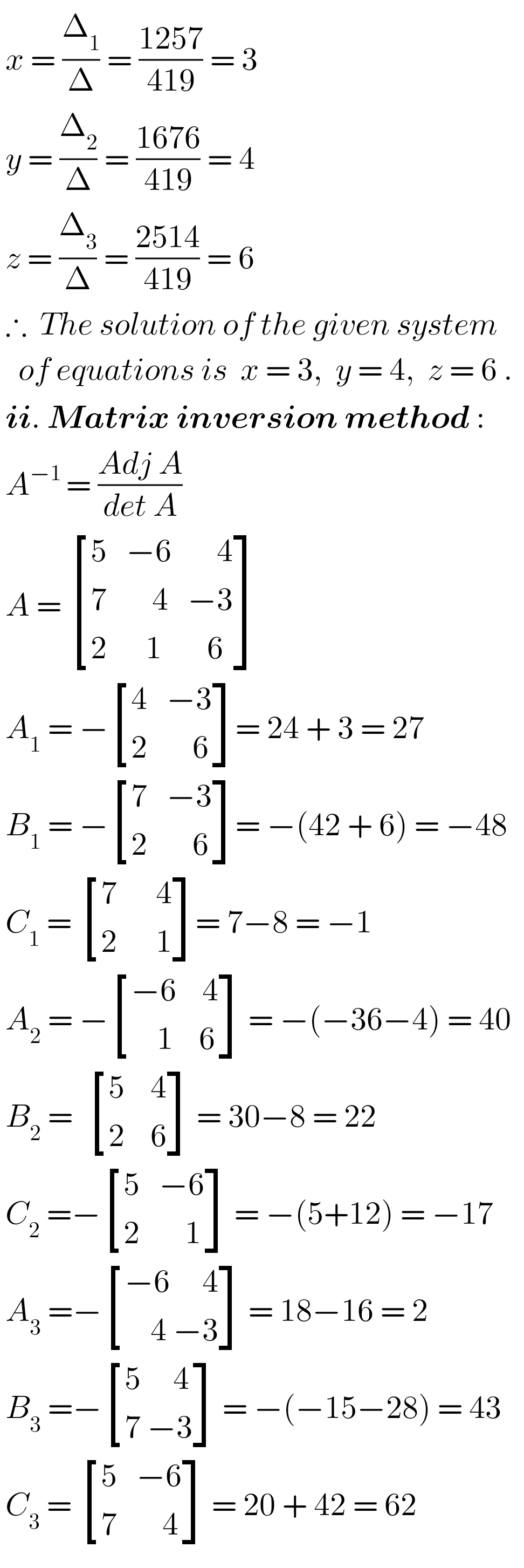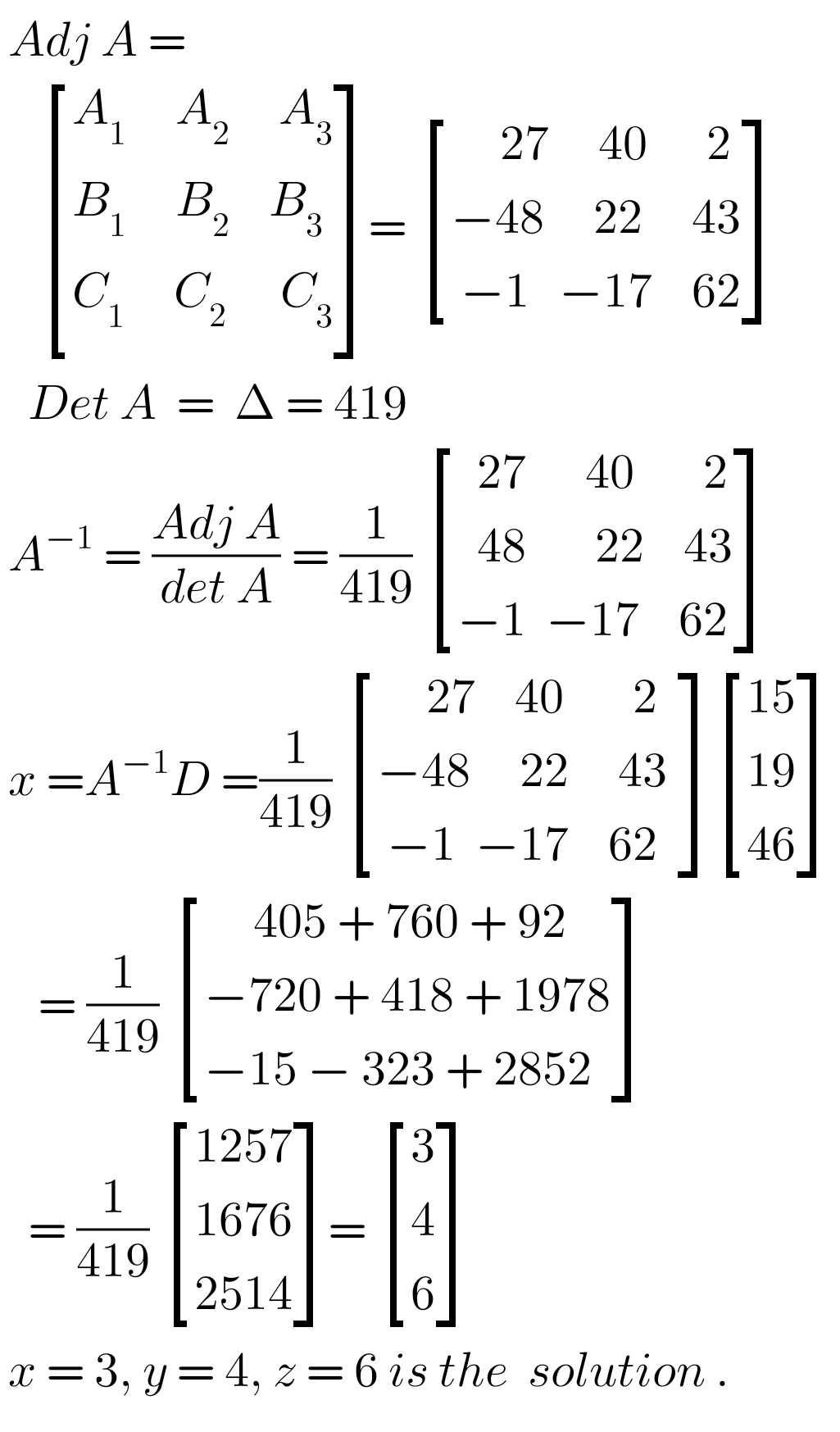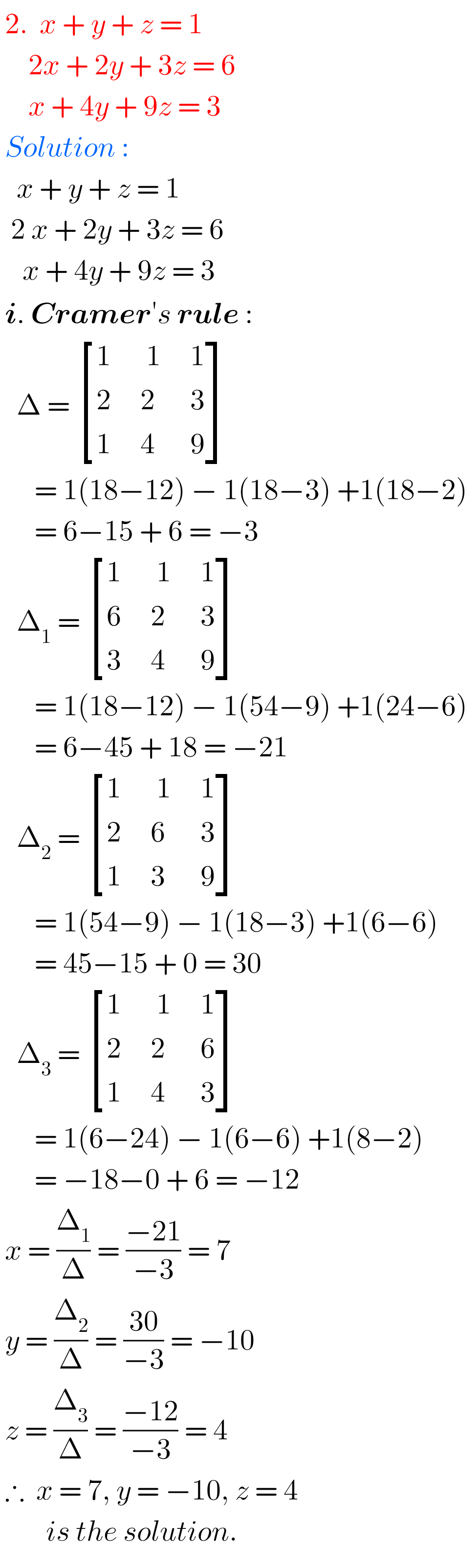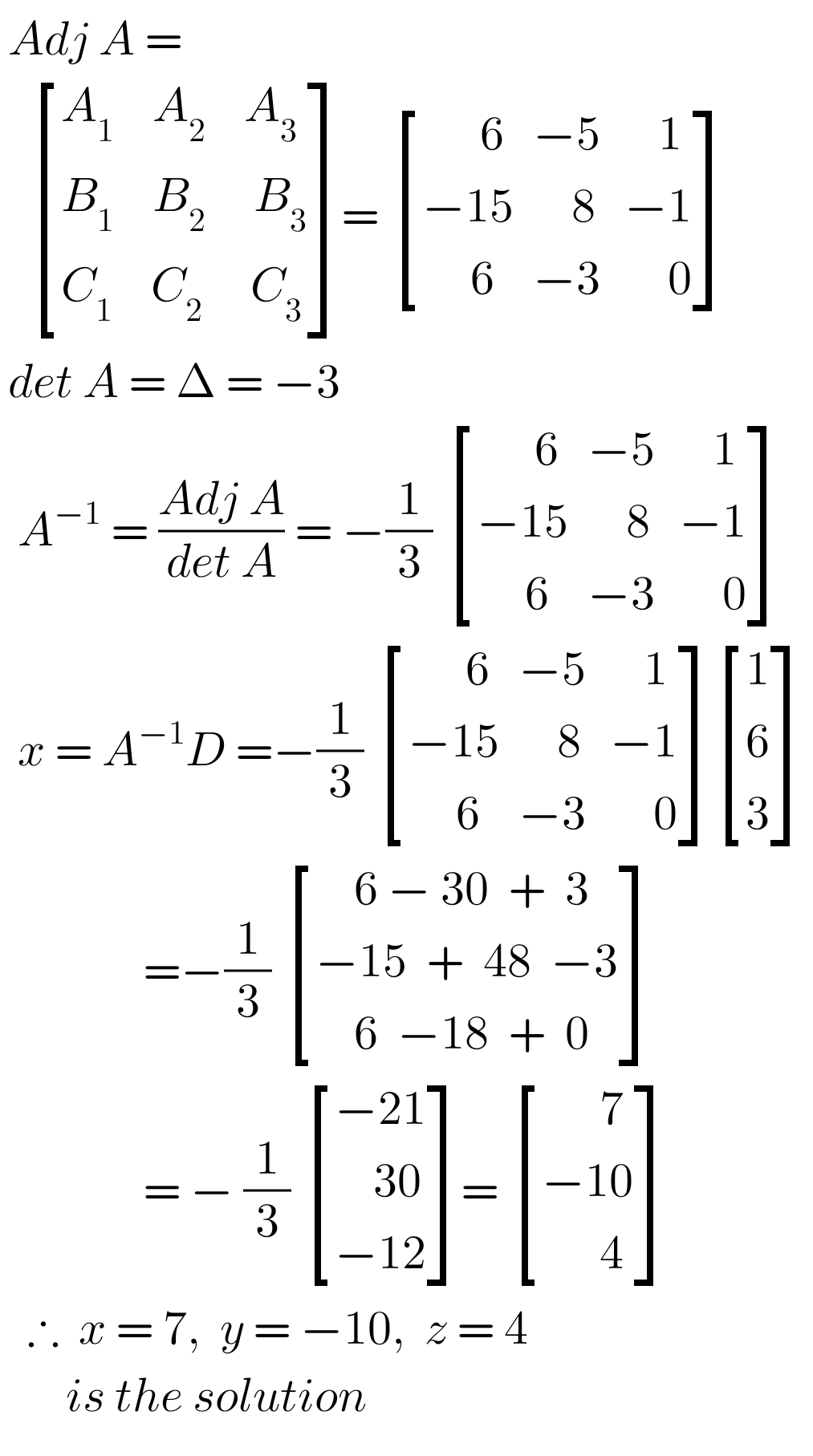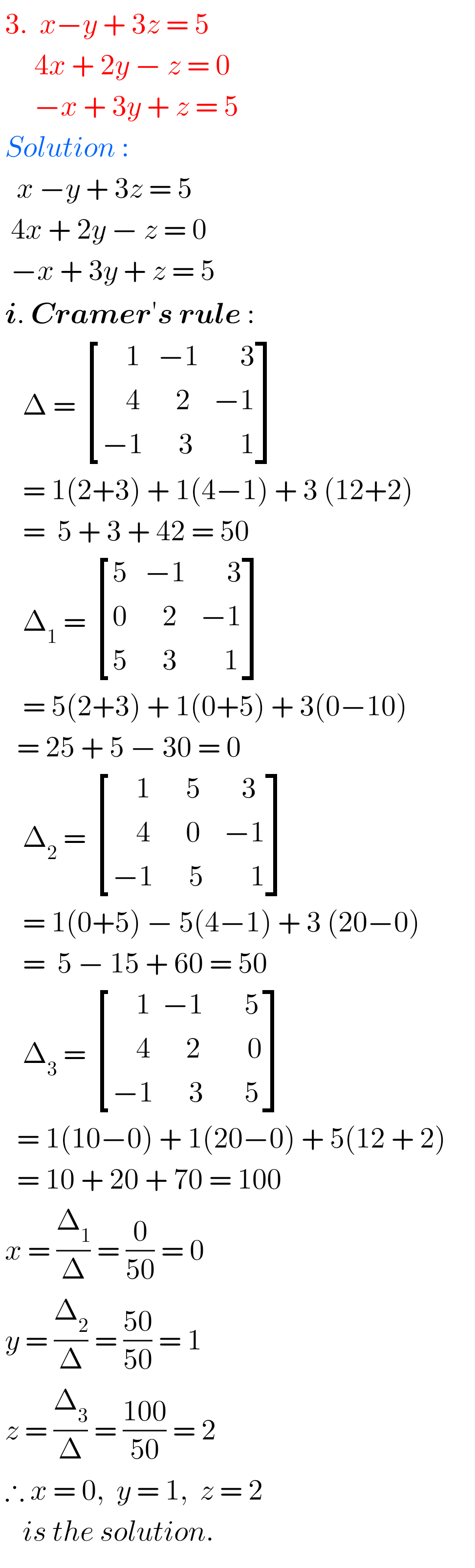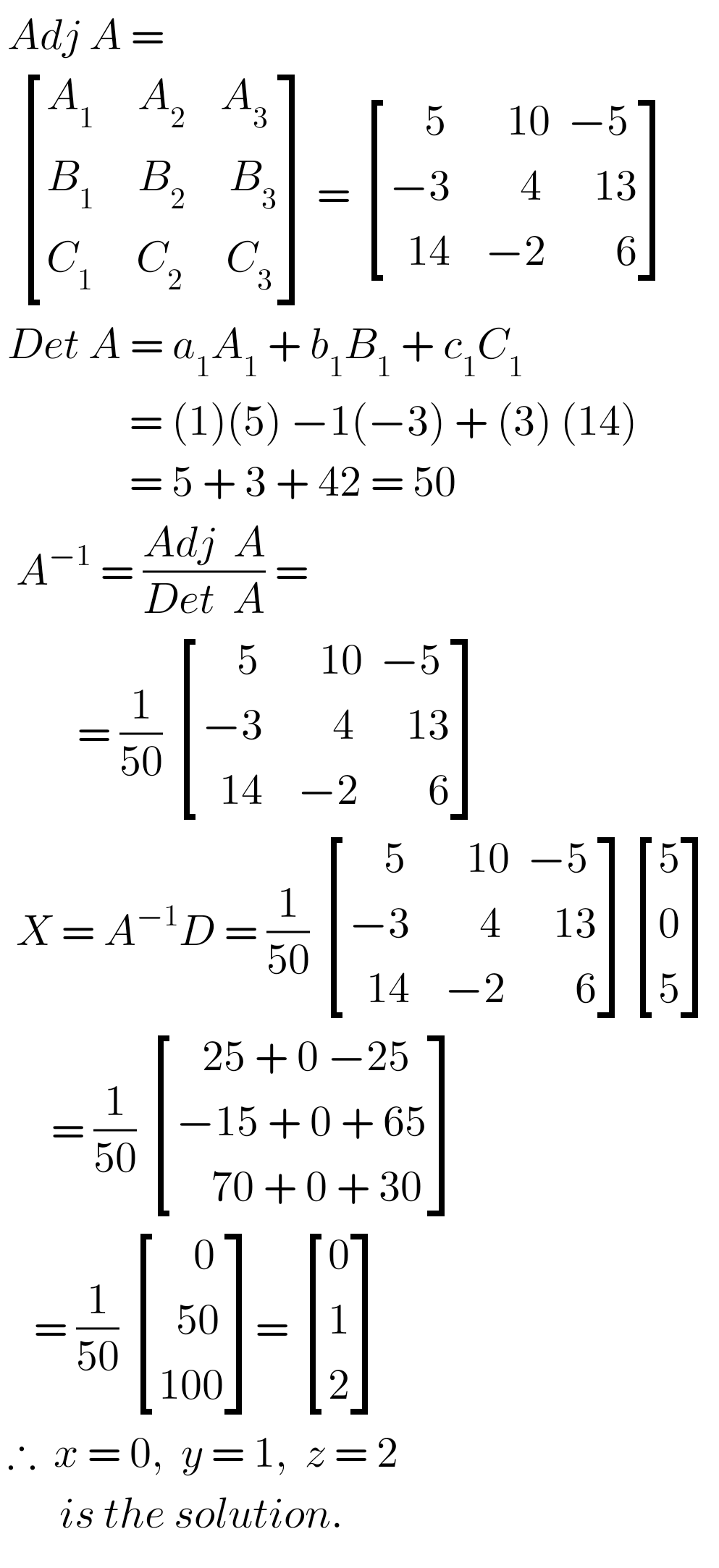## Maths 1A Solutions for Matrices Exercise 3(h)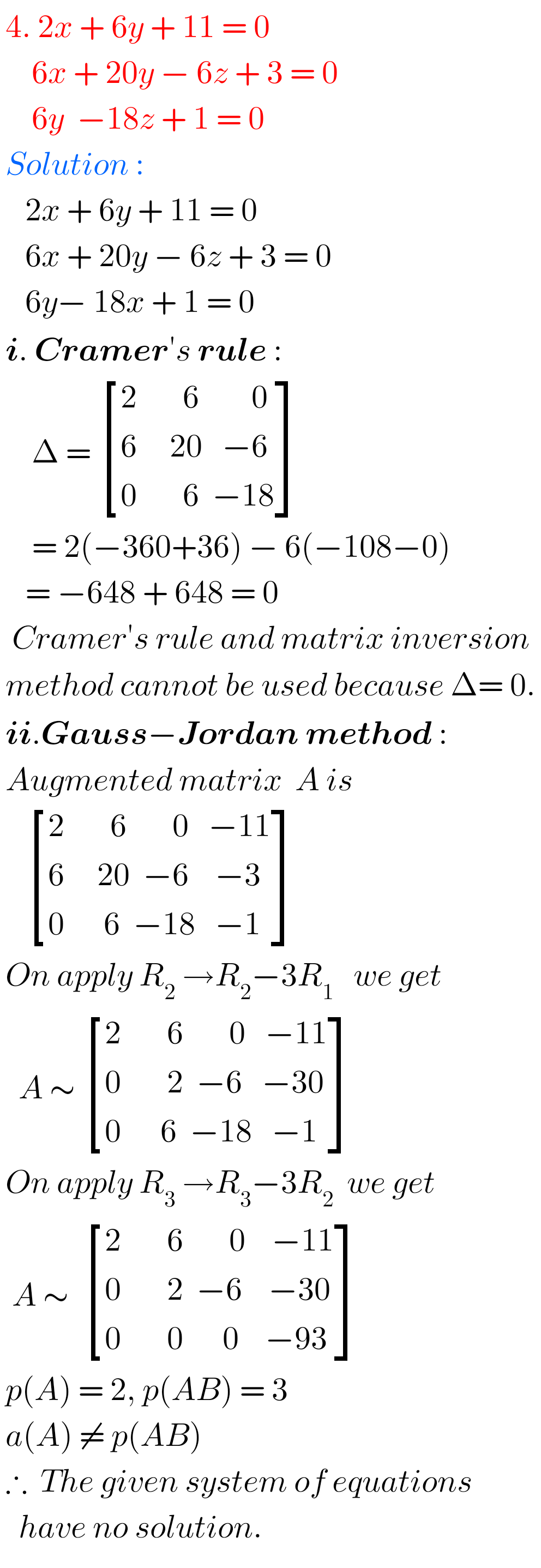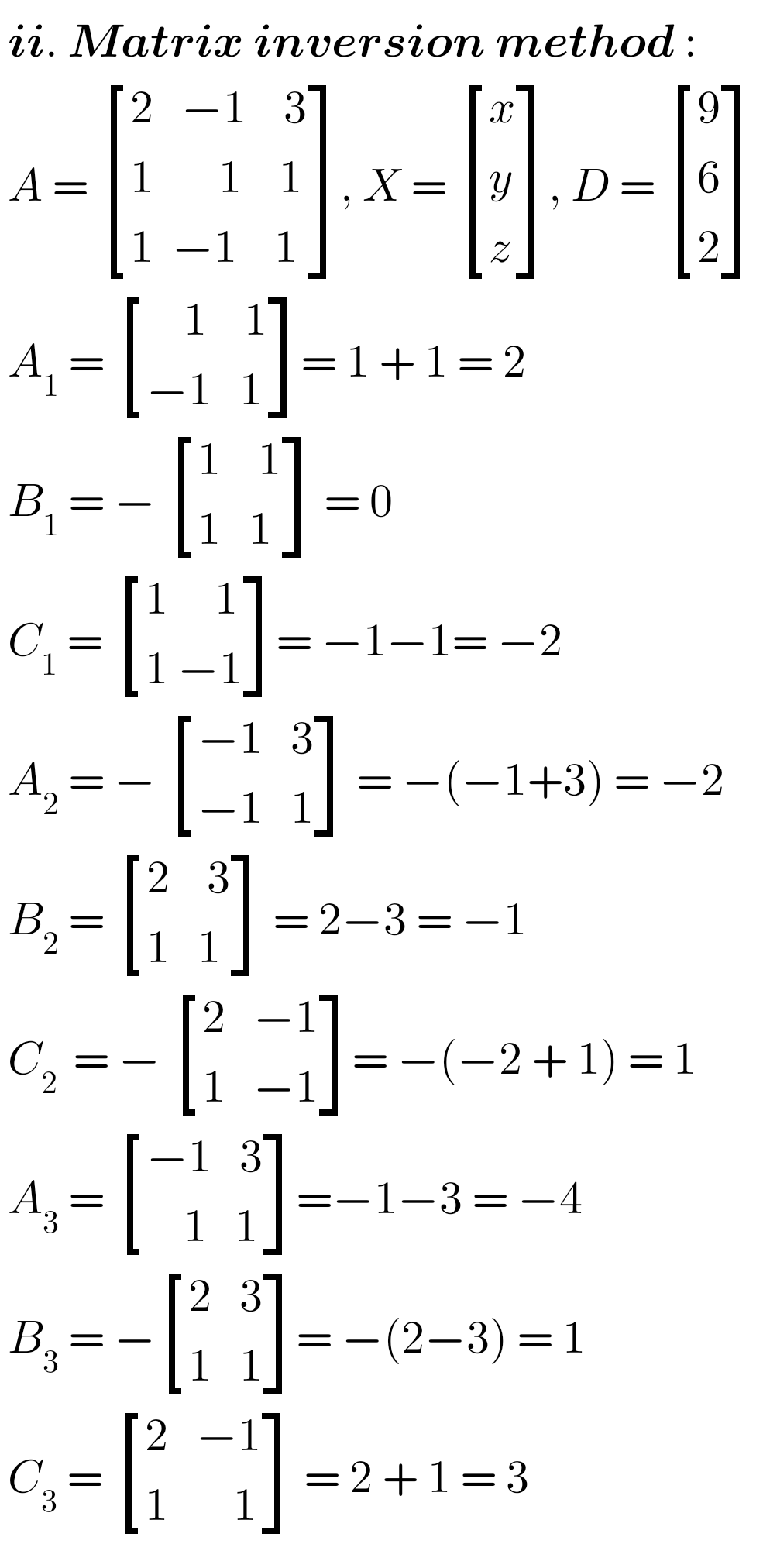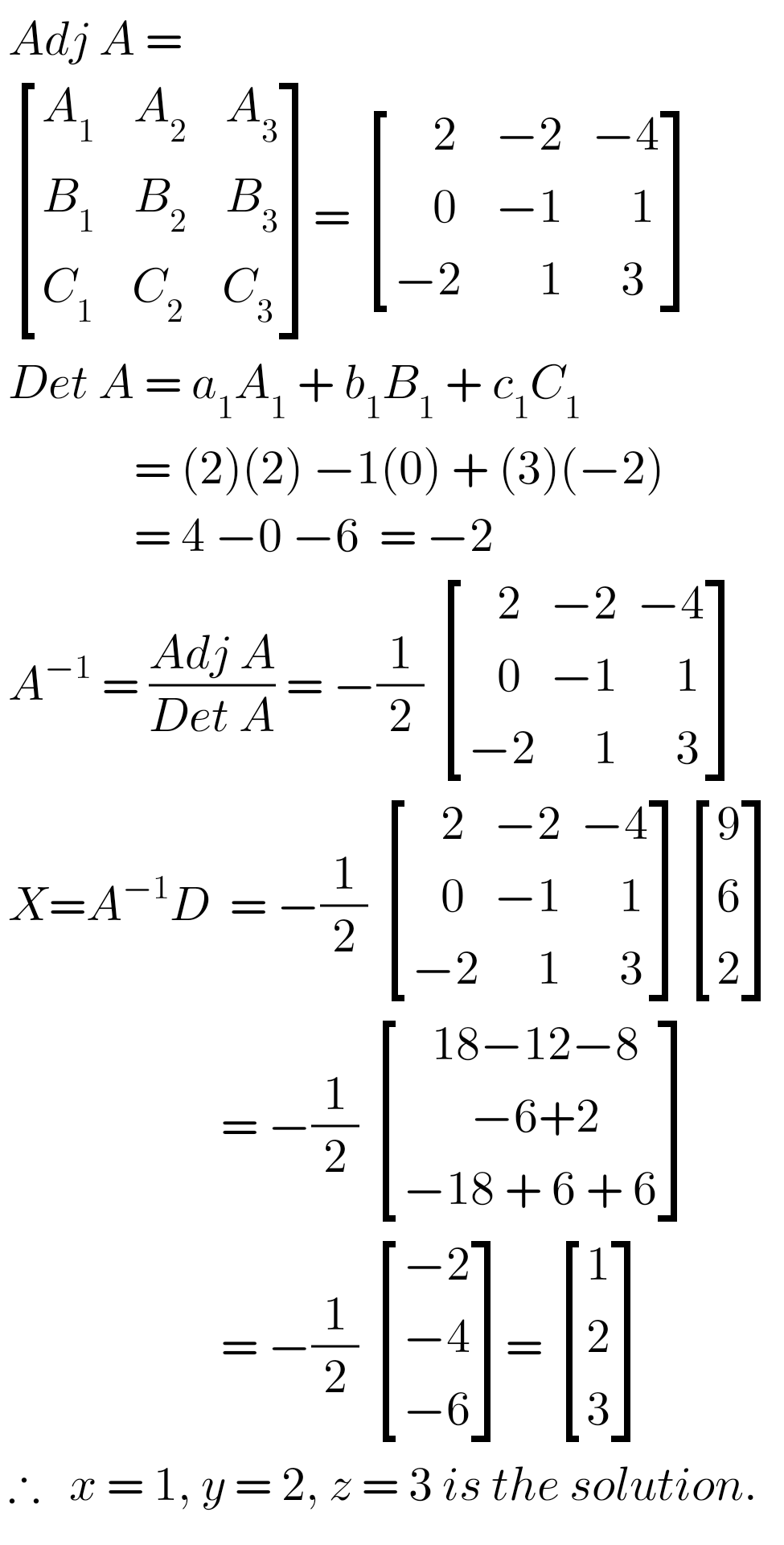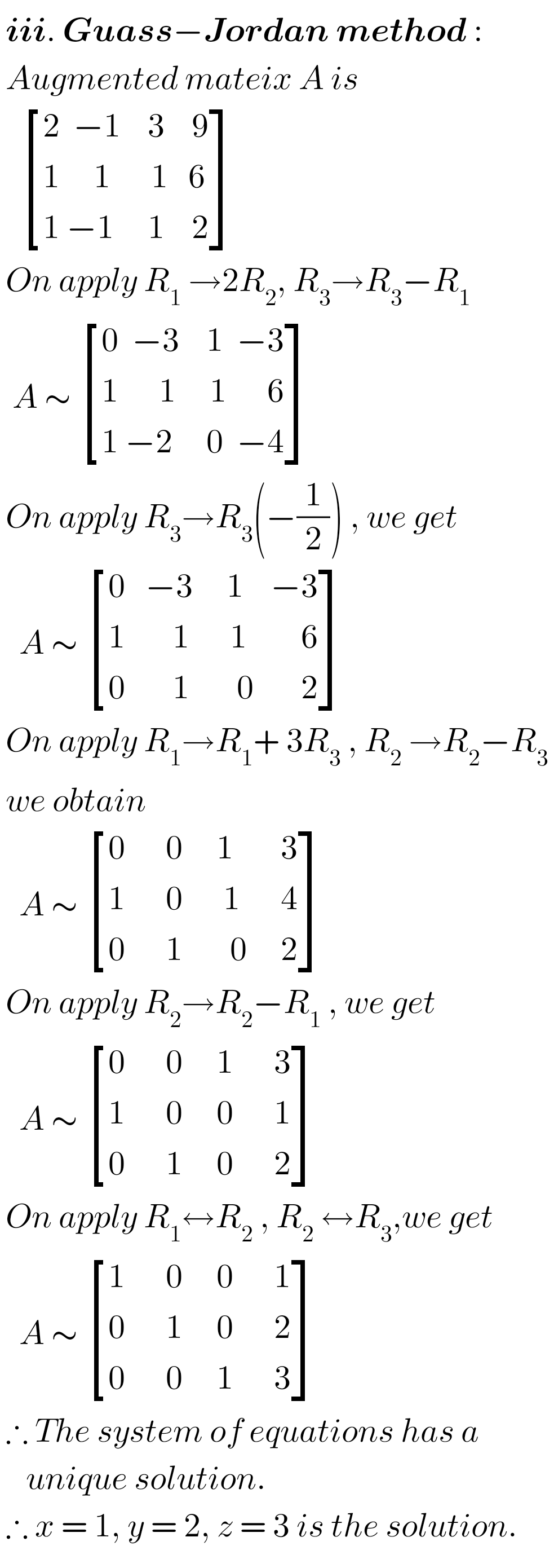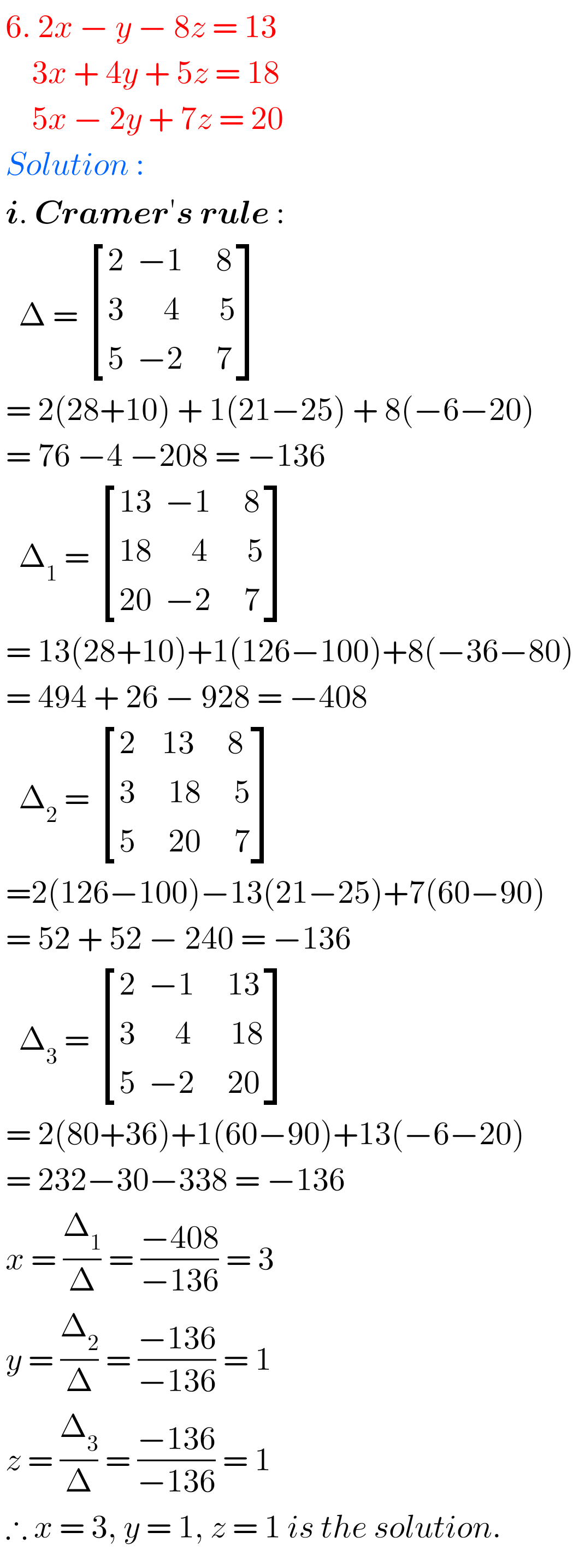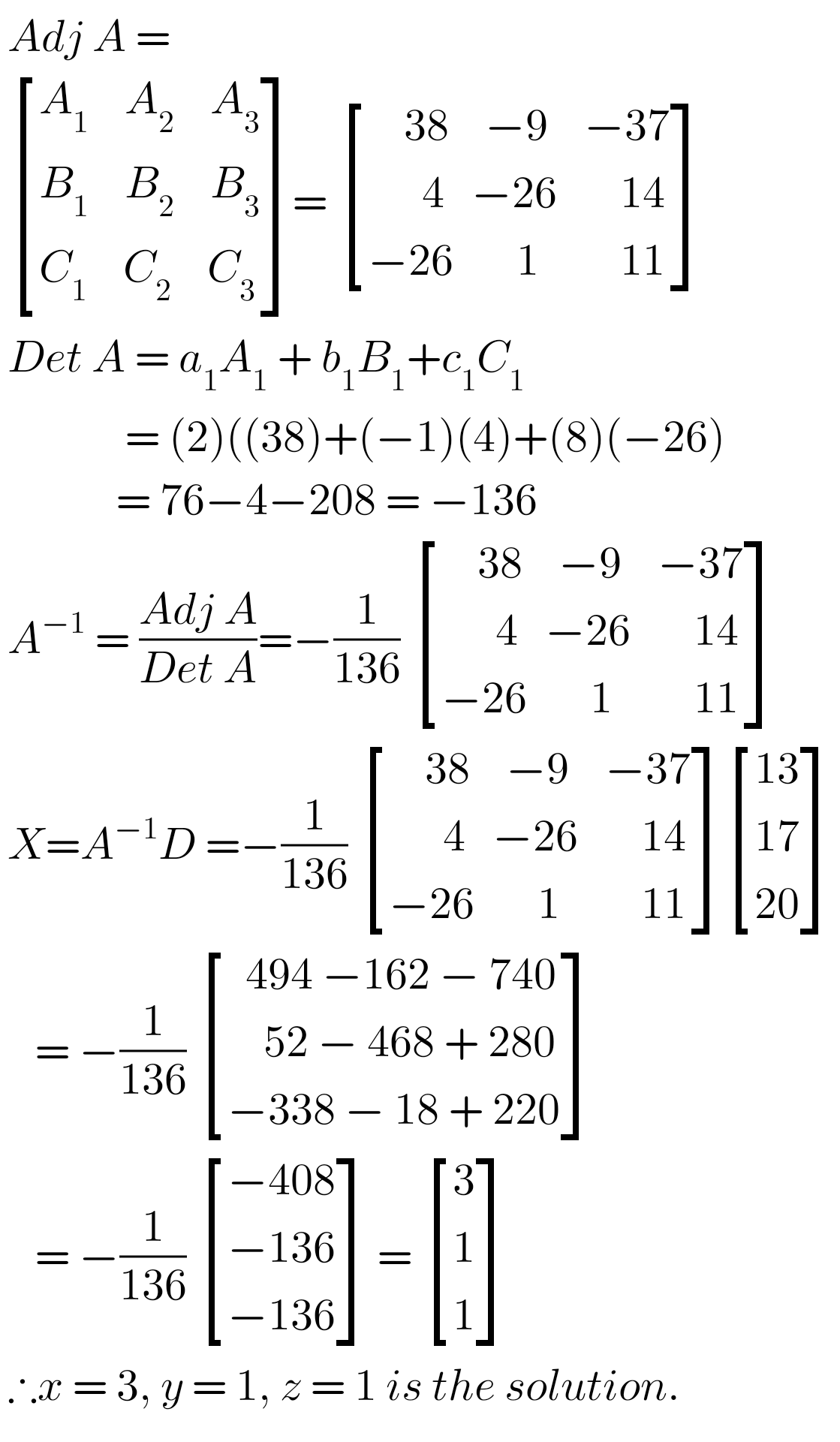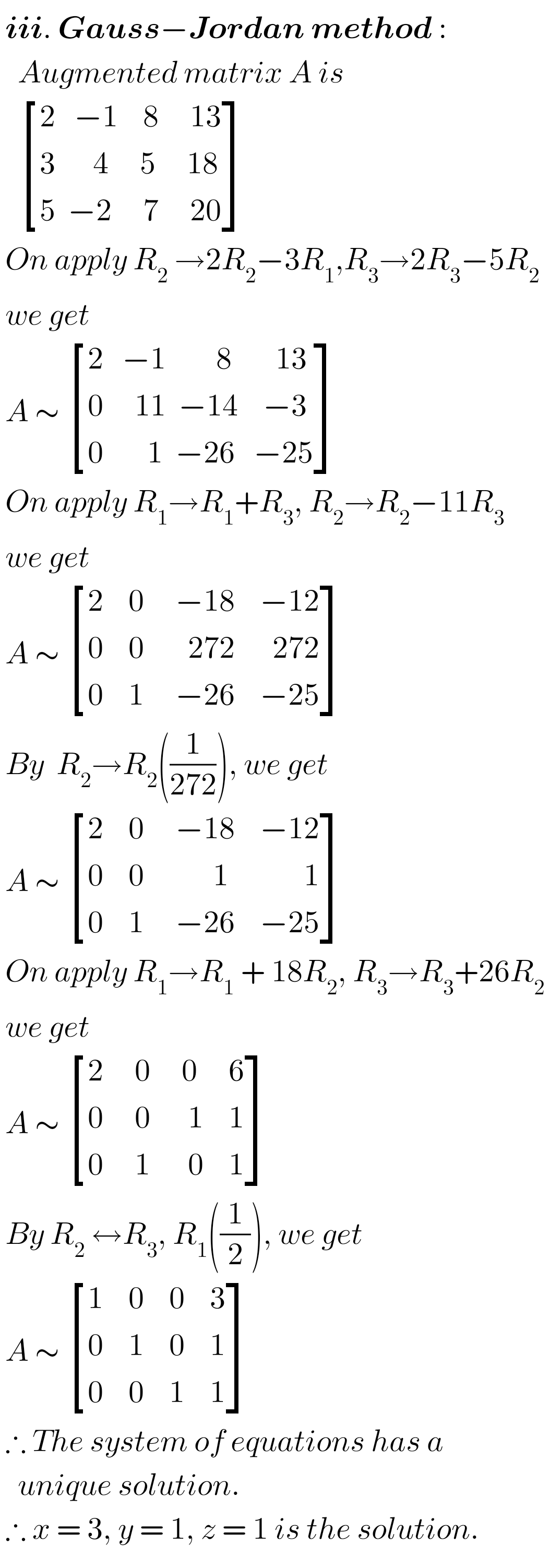## Matrices solutions exercise 3(h) Inter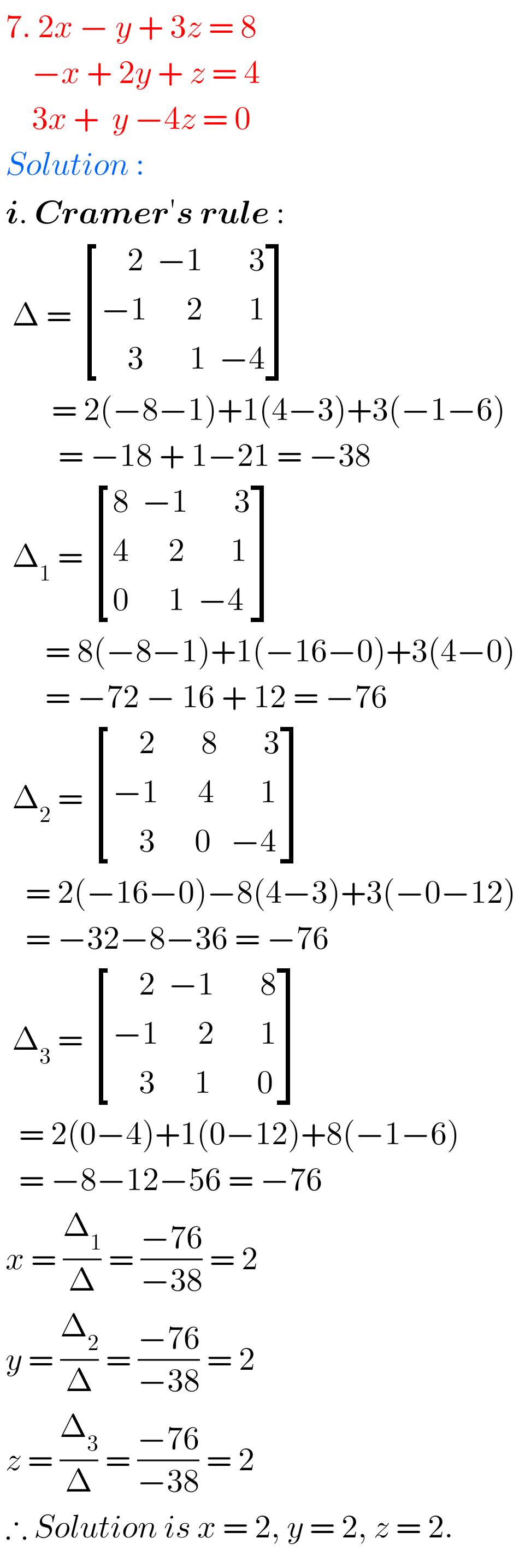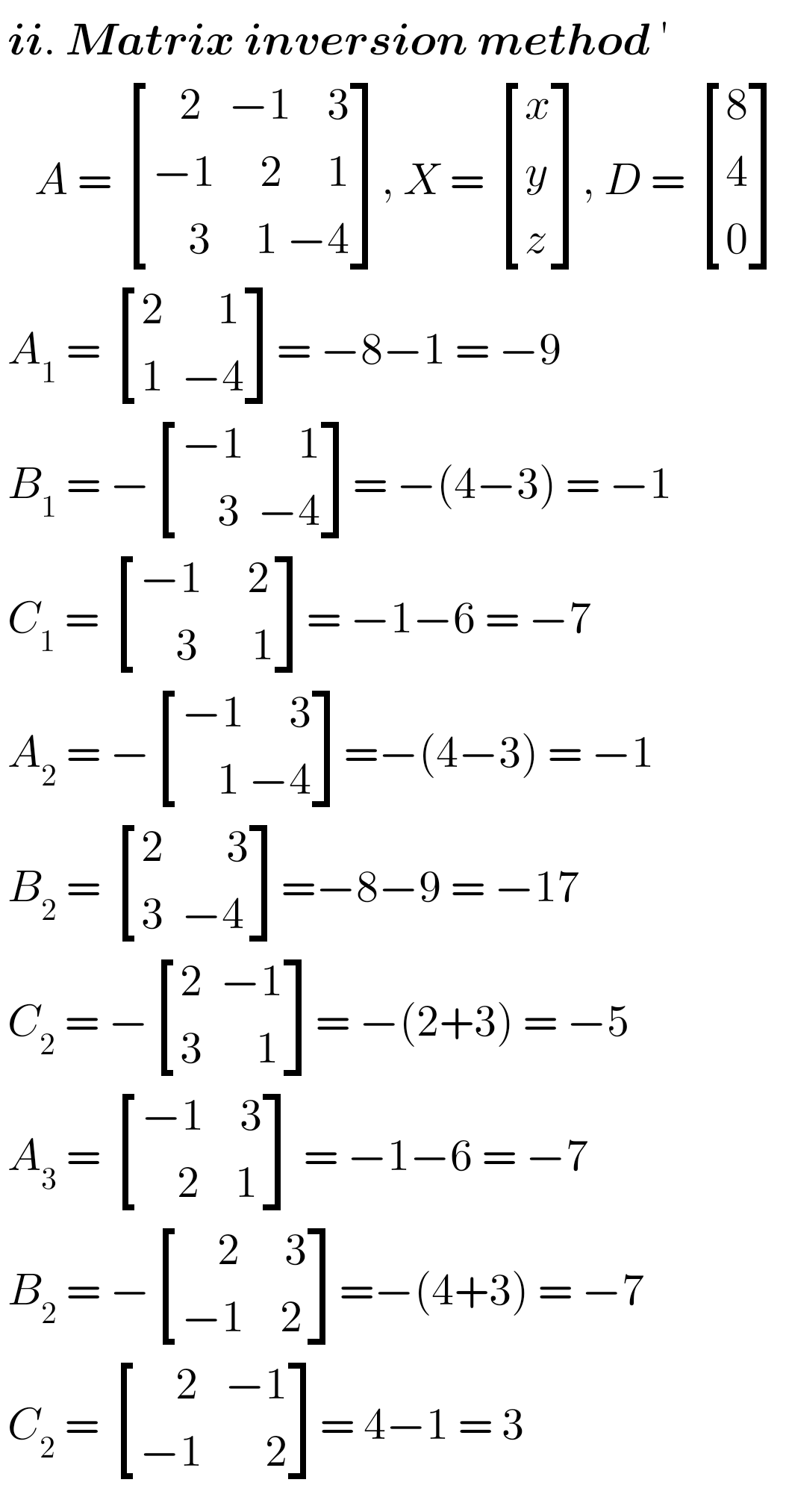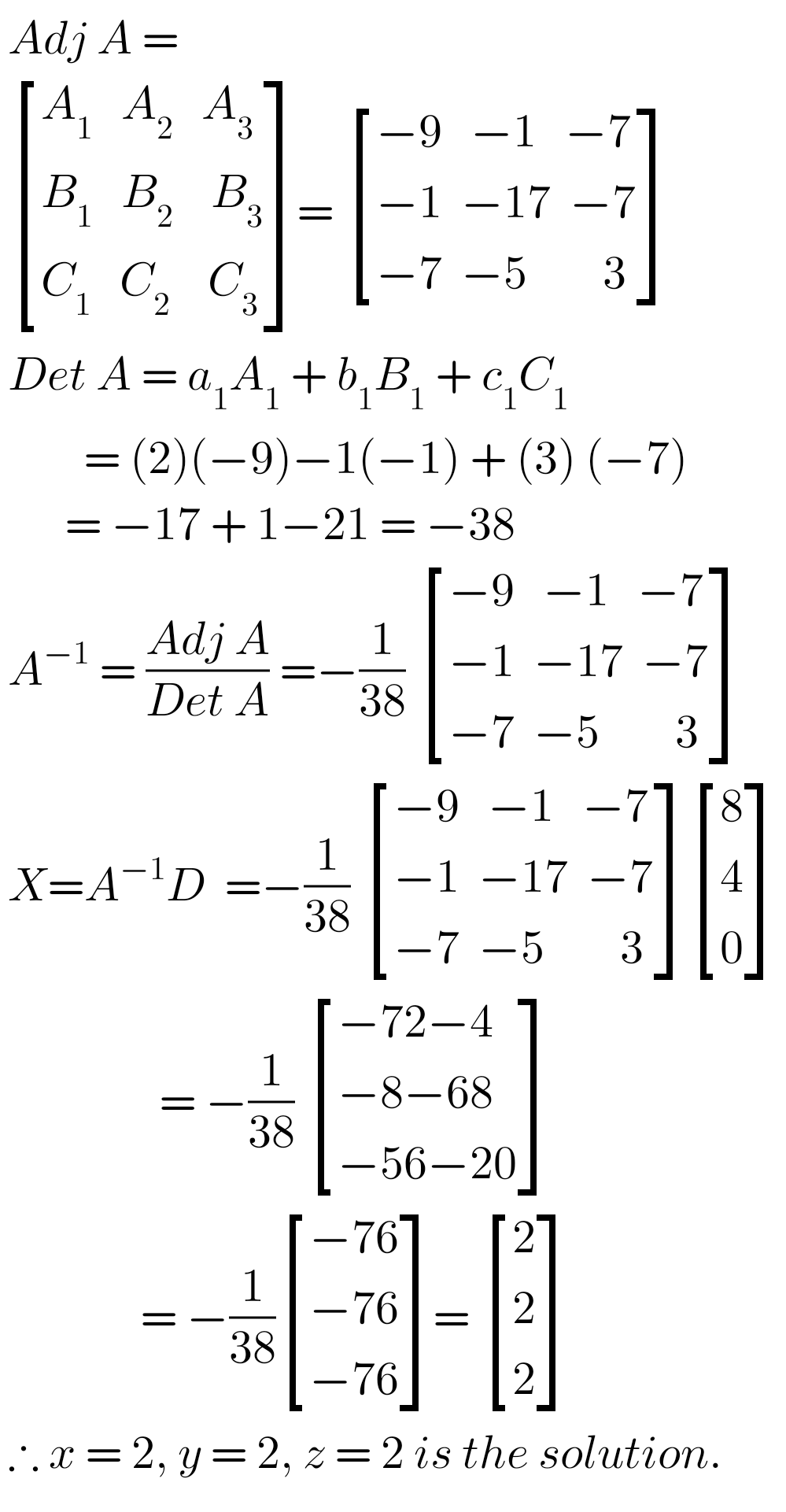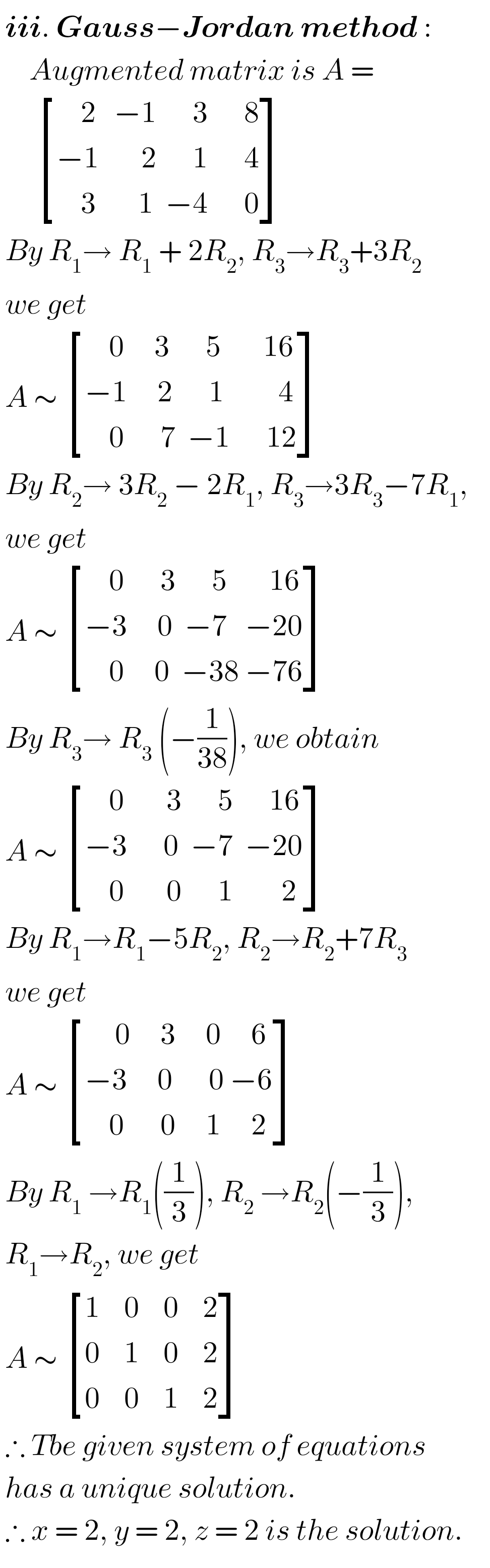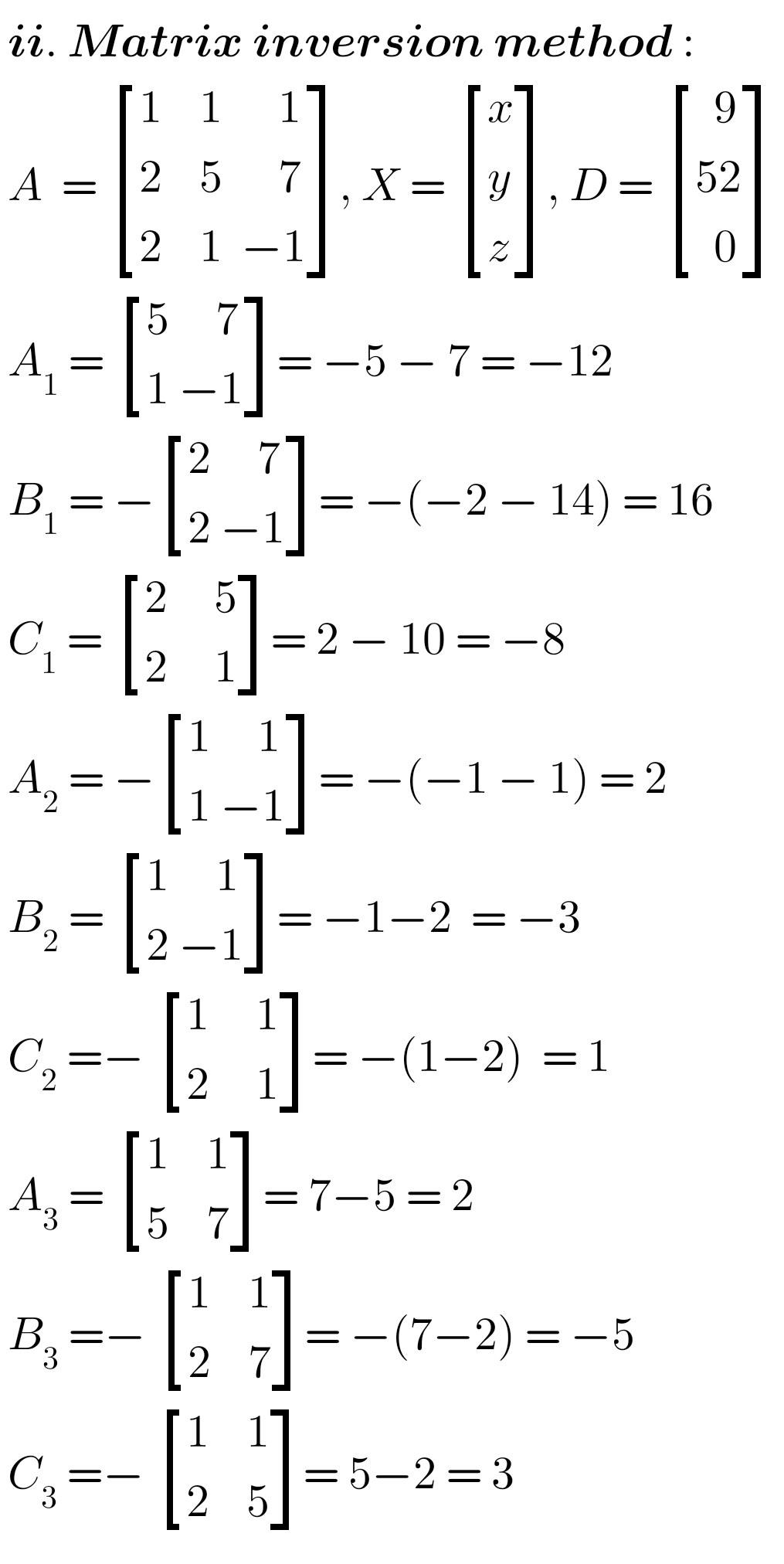Note : Observe the solutions and try them in your own method.

You can also see

SSC Maths text book Solutions class 10

Inter Maths 1A solutions

Inter Maths 1B solutions

Inter Maths 1IA text book solutions

Inter Maths IIB text book solutions

You can see the solutions for junior inter maths 1A for examination purpose

2. Matrices

Junior inter 1B solutions of Examination purpose

1Locus

Straight lines sa

Straight lines la

12. Rate measure